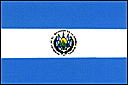El Salvador  [ Back to Seismic Design Code Index ]   [ Back to Country Index ]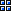SELECTION OF ANALYSIS METHOD FOR LATERAL FORCES

1. Static Lateral Force Method can be used for:

• Regular structures lower than 70 m of height, except those which are located in S4 soil type and their fundamental period is higher than 0.7 seconds.
• Irregular structures not having more than 5 stories or lower than 20m of height. Structures with irregularities A, B, or C according to table 4, or any other irregularity not defined in table 4 or 5, should also comply with section 3.8.1 to use the static lateral force method.

2. Dynamic Analysis of Force Method can be used for:

Any structure can be designed using this method. It has to be used specially for the cases in which the static lateral force method can not be used.SELECTIONSTATIC LATERAL FORCE METHOD FOR LATERAL FORCES

The base shear should be calculated using the following equation: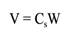Where Cs needs to be computed using: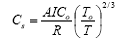The fundamental period (T) of the structure needs to comply with: To < T < 6To

Where: A: Area factor (see table 1)

I: Building importance factor (see table 3)

Co and To: Site coefficients (see table2)

T: Period of structure

R: Reduction factor (see table 6)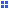Period of the structure

• Method A: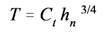• with:     Ct = 0.085 for ductile steel frame structure.
•             Ct = 0.073 for ductile RC frame structure.
•             Ct = 0.049 for every other structure.

• Method B: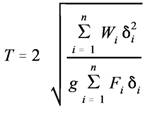With: Fi = Any appropriate approximation of force distribution.
* T using this method should not be less than 80% of T using method A.Vertical Distribution of Lateral Force

The force acting in every floor, including the top floor is evaluated using: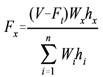Where the value of the extra force at the top Ft is: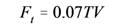Where: T = Using Method A
Ft = 0 when T < 0.7 sec
Ft < 0.25VHorizontal Distribution of Lateral Force

• Accidental Torsion Moment

Mt = (Fx)x * e

Where: (Fx)x = Seismic force for story x

e = 0.05 * Building dimension perpendicular to x axis.

• Increasing of Accidental torsion due to irregularities listed in table 5

When Torsional irregularities are present we need to increase:

The accidental torsion using factor Ax.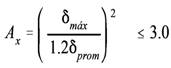The Base shear in the analysis direction using Bx:Story drift calculation and limits.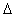= (Total displacement of story n+1) - (Total displacement of story n)

The total displacement of a story is calculated using: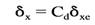Where: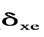= Elastic displacement of a story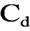= Increasing Displacement Factor

*The story drift calculated by this equation should not be larger than the allowable story drifta listed in table 7. For this calcualtion the fundamental period can only be estimated using the B method, and the limitation of only taking the 80% of the period calculated using method A shold not be taken into account.Vertical component of seismic Forces.

• Horizontal cantilever members should be designed to resist a net upward force equivalent to 0.5 A times the dead load, in addition to the other combinations of applicable loads.
• Horizontal prestressed members should be designed, in addition to all the combinations of applicable loads, using no more than the 50 percent of dead load for gravitational loads, alone or in combination with the effect of lateral forces.DYNAMIC ANALYSIS FOR LATERAL FORCES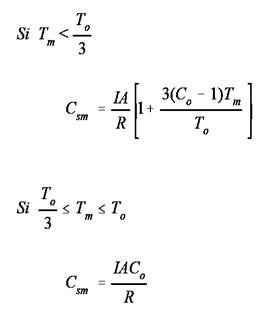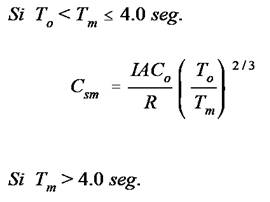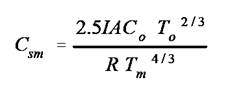Response Spectrum Analysis

1. Number of Modes to use: As much as needed to achieve 90 % of participant mass.
2. Mode combination: Most danger combination should be used.
3. Results evaluation: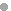Irregular st.: 100 % of static base shear.Regular st.: 90% of static base shear using T from Method A. But greater than 80% of static base     shear using Method B.
4. Directional Effects: Take into account the ortogonal effects.
5. Torsion: Take into account torsion effects.Diaphragms.

The story deck for every floor should resist a force equal to: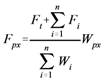Where: 0.35 A I Wpx < Fpx < 0.75 A I WpxOrtogonal Effects

To take into account the effect of seismic forces acting perpendicular to the analysis direction we can use:

(Mtx)t = 100% Mtx + 30 % Mty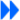Click here for PDF file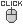Go back to the top of the page